# 一文搞懂仿射变换原理及Python实现代码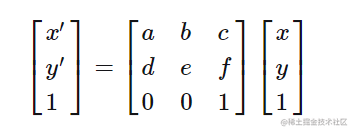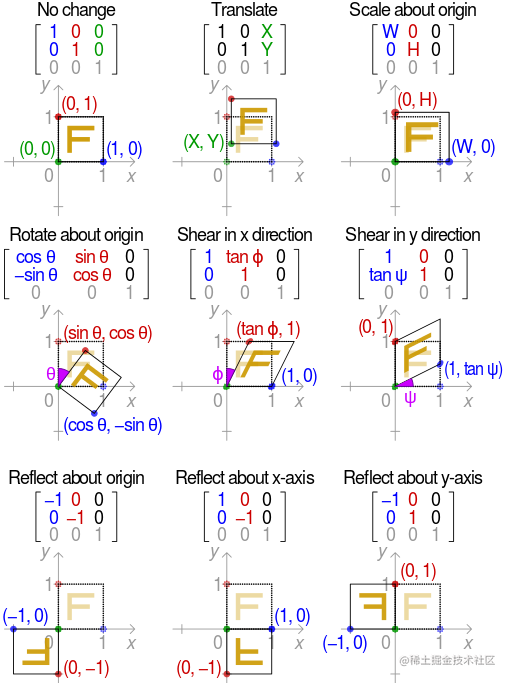## 1. cv2.getRotationMatrix2D详解

opencv的cv2.getRotationMatrix2D函数可以获取旋转变换矩阵。输入中心点坐标(centerX,centerY)，旋转角度θ和缩放比例1，给出M变换矩阵： $$\left[ \begin{matrix} cosθ & -sinθ & (1 - cosθ) * centerX + sinθ * centerY \ sinθ & cosθ & (1 - cosθ) * centerY + sinθ * centerX \ 0 & 0 & 1 \end{matrix} \right]$$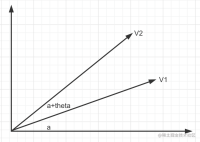V1点和原点连线与水平线夹角a，V2点和原点连线与水平线夹角b=a+θ。计算旋转变换矩阵： 记V1=(x1, y1), V2=(x2, y2)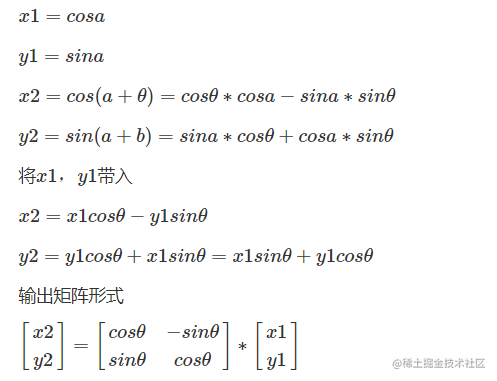1.先把旋转点平移到原点
2.然后进行以上旋转操作
3.按1的逆操作平移回去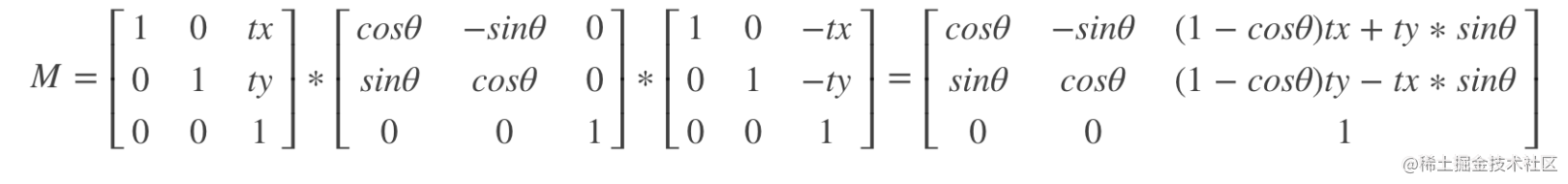## 2. warpAffine操作

### 2.1 获取M矩阵进行仿射变换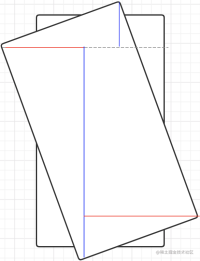### 2.2 扩大画布

M[0,2]+=(new_W−w)/2

M[1,2]+=(new_H−h)/2

## 3. 图像仿射变换代码

Talk is cheap, show me the code. 直接上代码： python def affine_transform(image, angle, scale=1.0): h, w = image.shape[:2] center_x, center_y = width // 2, height // 2

M = cv2.getRotationMatrix2D((center_x, center_y), angle, scale)
cos = np.abs(M[0, 0])
sin = np.abs(M[0, 1])

new_h = int(h * cos + w * sin)
new_w = int(h * sin + w * cos)
M[0, 2] += (new_w − w) / 2
M[0, 1] += (new_h − h) / 2

new_image = cv2.warpAffine(image, M, (new_w, new_h))
return new_image




## 4. 单点仿射变换代码

M = cv2.getRotationMatrix2D((center_x, center_y), angle, scale)
cos = np.abs(M[0, 0])
sin = np.abs(M[0, 1])

new_h = int(h * cos + w * sin)
new_w = int(h * sin + w * cos)
M[0, 2] += (new_w − w) / 2
M[0, 1] += (new_h − h) / 2

new_points = np.dot(M, points.T).T
return new_points